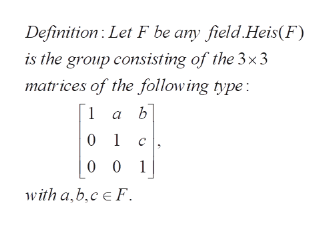Let Heis(F) be the Heisenberg group over the field F with the group operation being matrix multiplication. Show that if F is finite of order q then the order of Heis(F) is q3; conversely show that if F is infinite then Heis(F) is infinite.

Question
1. Let Heis(F) be the Heisenberg group over the field F with the group operation being matrix multiplication. Show that if F is finite of order q then the order of Heis(F) is q3; conversely show that if F is infinite then Heis(F) is infinite.

Step 1

To establish tha tthe group  Heis(F) has the  required orders, when F is finite or infinite

Step 2

Definition of the group Heis(F), where F is a field.help_outlineImage TranscriptioncloseDefinition Let F be any field Heis(F) is the group consisting of the 3x 3 matrices of the follow ing type: 1 a b 0 1 C 0 0 1 ith a,b,c F fullscreen
Step 3

If F is a finite field consisting of q elements, a,, b  and c take any of the q values in F independently;. As each of them can assume q values, the ...

Want to see the full answer?

See Solution

Want to see this answer and more?

Our solutions are written by experts, many with advanced degrees, and available 24/7

See Solution
Tagged in

Math